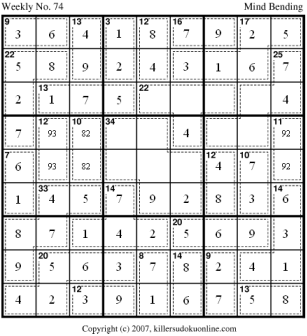Approach to Weekly No. 74

Here is an approach to weekly 74. This puzzle is one of my favorites because it combines an unusual application of the rule of 45 and some math in addition to traditional sudoku logic.

 First, fill in the obvious cages.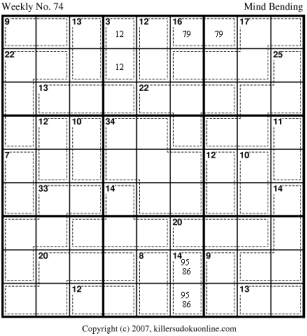Using the already filled in 16-cage on the top row we can narrow down the possibilities for the 13-cage in R89C3 and the 12-cage in R89C5.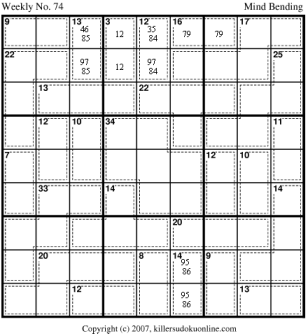The 17-cage in the top right has only two possible sums: 12347 and 12356. Combine this with the 3-cage in R89C4 eliminates using a 1 or 2 in any other cells in the top two rows. Thus, the 9-cage in R9C12 has only two possible sums: 45 and 36.The only place to place 1 and 2 in the top left nonet is in row seven. The 13-cage in R7C234 cannot have both the 1 and 2. So, R7C1 must be 1 or 2.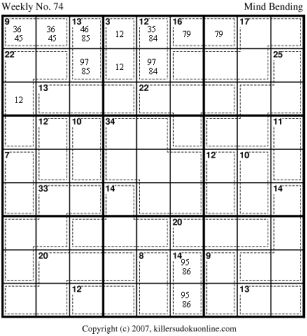The rule of 45 indicates that the blue region sums to 20. The possible sums for this are: 389, 479, 569, 578. We can eliminate 569 as a possible sum since a 6 cannot appear in R7C12 (due to its use in either the 9-cage or the 13-cage). And, if it were in R7C9, then 95 would be in R7C12 which also doesn't allow a solution to the 9- and 13-cages in that nonet. This means that the 6 in row seven must appear in the 17-cage. So, we know that the 17-cage must be 12356. Furthermore, R9 of the 17-cage must contain a 1 or a 2 AND 3 or a 5.Since the 9-cage in R9 must have either a 3 or 5 AND the R9 part of the 17-cage must have either a 3 or 5, we can eliminate 3 and 5 from the rest of row 9.Now, we must apply the rule of 45 several times to come up with relationships between a collection of cells. First, the blue cells must sum to 16. The red cells must sum to 12.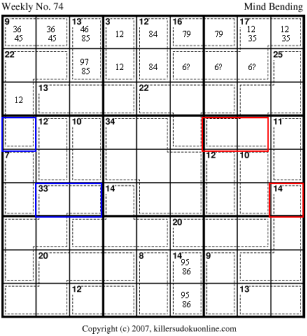The blue cells here must sum to 11.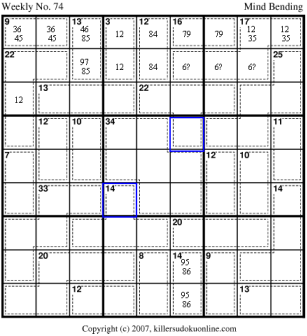The blue cells here must sum to 12. The red cells here must sum to 11.Now, the tricky application of the rule of 45. The blue cells must sum to 18. The red cells must sum to 16. Finding this application of the rule of 45 is the key to this puzzle.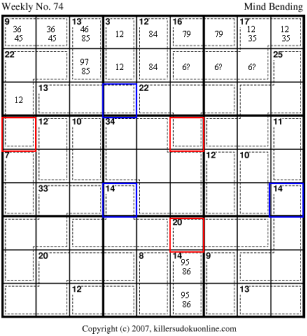Now we have 7 equations and 8 unknowns. So we can't get exact solutions quite yet, but we can narrow down the ranges of the cells. We have: A+B+C = 22 D+E+F = 17 A+D = 16 B+E = 11 C+F = 12 G+E+D = 16 H+B+C = 18 From this we can derive: F-G = 1 A-H = 4Lets consider A (in blue) and H (in red). A is part of a 33-cage with 5 cells. Thus, A must be at least 7. Since A=H+4, it cannot be more than 13. So, A is between 7 and 13. And, H is between 3 and 9. Actually, it cannot have 4 or 8 either so it is limited to 3,5,6,7, or 9. Remember that H+D=12, where D is R6C1. So, D must be 9,7,6,5 or 3.Before we eliminate some of the possible values in R6C1, lets look at the 7-cage (in blue). We know that the red part of the 33-cage cannot have a 1 or 2. So, the 1 and 2 must be the 7-cage and the 10-cage.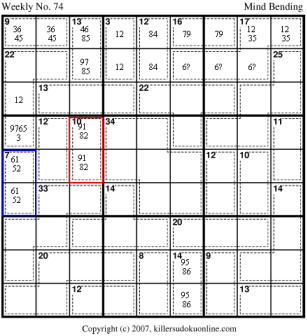We can now eliminate some of the values in R6C1 (in red) based on the the blue region which we know must sum to 20. 3 is not possible in R6C1 because 3+1=4 which leaves over 18 in R7C12, which can't be done with 2 cells, and, 3+2=5 which leaves over 17 in R7C12 which would put a 3 in R8C9. But, 3 is already used in the upper right nonet.9 is also not valid in R6C1 because it would leave 7 for the blue region (R4C23). However, a 7 in R4C23 leaves 26 left for the remaining three cells in the 33-cage which is not possible. We can now fill in the corresponding values in R7C4, since we know that R7C4+R6C1 must sum to twelve.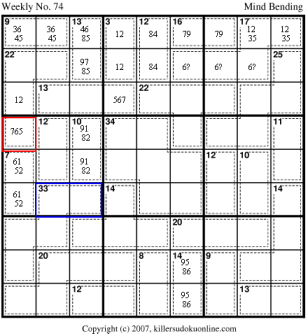Given the values already filled in for the middle left nonet we can deduce that either 3 or 4 must be in the 12-cage and a 3 or 4 must be in the blue region (R4C23). So we can fill in the possibilities for the 12-cage.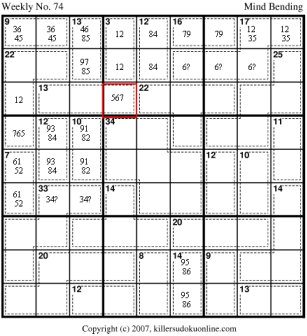Lets look at the 13-cage in the upper left nonet. The possible sums, given the values in R7C4 (in red) and the fact that the portion of the 13-cage in the nonet must have either a 1 or 2 are: 157 (with 157 possible in the nonet) 247 (with 24 in the nonet) 256 (with 25 in the nonet) We can eliminate 15:7 (with the colon dividing the sum between inside the nonet and the red cell) because it requires the 22 cage to be 5287, which puts a 5 in R8C9 which is illegal. Similarly we can eliminate 25:6 because it requires the 22-cage to be 6187 which also requires a 5 in R8C9. So, the sums that are still legal for the 13-cage are: 17:5 24:7 which eliminates 6 as a possible value in R7C4 and R6C1.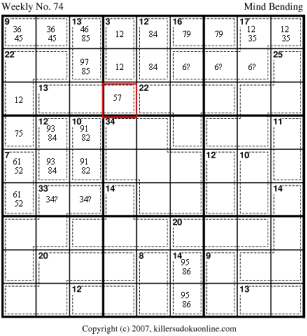Now we can see that the 7-cage in R45C1 must be 61. And then we can clean up a bit.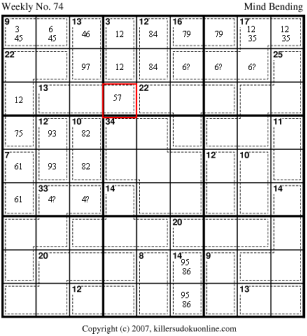Now we can eliminate the other sum for the 13-cage because the 9-cage (in red) and the 13-cage (blue) both use either 4 or 6. So the only valid sum for the 13-cage on row seven is 175.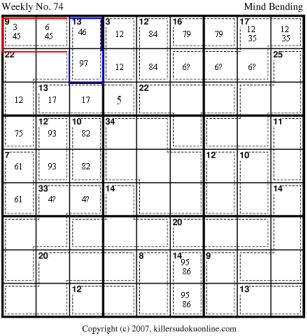Cleaning up a bit ...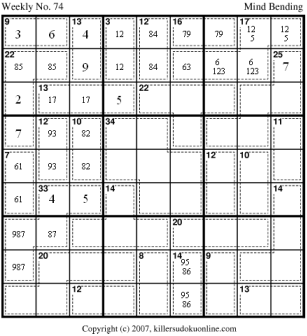R1C1 must be a 4. This requires R8C1 to be a 5, which determines R3C2. Since there are already 5s in column three and four and there is a 4 in row one and a 9 in column 3 we can fill in the 12-cage on R1. This forces the 14-cage in C6 to be 86. Which forces R8C6 to be a 3.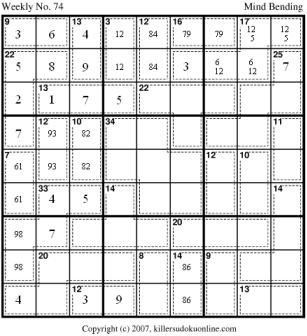R12C2 must be 52 which leaves the remaining 2 cells of the 20-cage summing to nine. Since one of those values must be a 1 or a 6 and there is an 8 in the nonet to the right, we know it must be 63. This determines the placement of the 8 and 6 in the 14-cage. Which then determines the arrangement of the 9 and 8 in the lower left nonet. The only possible sum for the 8-cage is 17.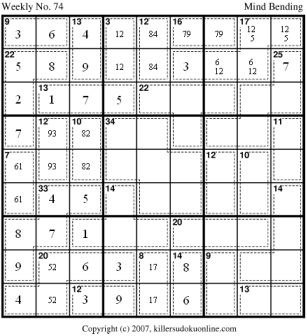R4C4+R6C6=11. Given the other values already in their rows and columns we can narrow the possible values down.Recall that the red cells must sum to 16. Thus, R3C6 must be either 4 or 5. Also recall that the blue cells must sum to 18, which means R4C4+R4C9=13. Given that R4C4 is 76, this means R4C9 is also 76. Which means no other 6 is allowed in the row.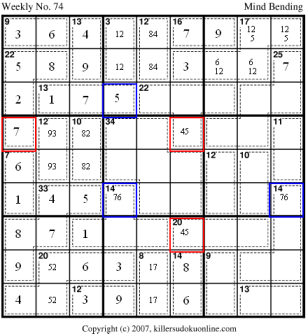Since there is already a 7 in row three we know that R3C6 cannot be a 4. The 7 in the row also requires the remaining cells to be 96. We can also set R6C6 to be 4.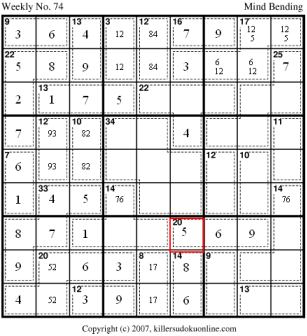A little cleaning up soon leads to this this and now almost every cell is a simple deduction. Good luck!